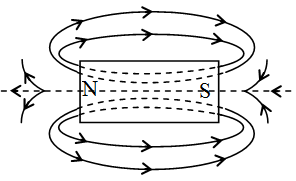Ex﻿

# 2. Magnetic Effect of Current

#### Magnetic Field

Magnetic Field Lines of Magnetic field (Magnetic lines of force)
Definition : A magnetic line of force, is a line straight or curved, in the magnetic field of a magnetic pole of magnetic dipole, such that the tangent at any point of this line gives the direction of the magnetic field at that point.
Properties of Magnetic Lines of Force
These have following properties :
They are always nearly normal to the surface of magnet at every point.They start from a north (positive) pole and end at a south (negative) pole.
Two lines of force do not intersect each other.
They tend to contract longitudinally (longitudinal contraction). They tend to expand laterally (lateral repulsion) so as to exert lateral pressure on neighboring lines.
(The above two properties are similar to that of a stretched rubber band).
The number of magnetic lines of force passing normally per unit area about a point, gives the intensity of the magnetic field at the point.

If you want to give information about online courses to other students, then share it with more and more on Facebook, Twitter, Google Plus. The more the shares will be, the more students will benefit. The share buttons are given below for your convenience.
×

#### NTSE Physics (Class X)

• Electricity
• Magnetic Effect of Curent
• Light Reflection
• Light Refraction
• Human Eye & Colourful World
• Source of Energy
• Motion

#### NTSE Physics (Class IX)

• Force & laws of motion
• Work and Energy
• Gravitation
• Sound

SHOW CHAPTERS